Question

a 10 kg block is attached to a light chord that is wrapped around the pulley of an electric motor. at what rate is the motor doing work when it is pulling the block upward w an instantaneous speed of 3 m/s and an upward acc of 2 m/s^2?

1.ngocdiep

The rate of work is 360 W

Explanation:

Given:

Speed of block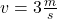Upward acceleration of block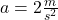Mass of block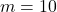kg

First Find the force act on block due to gravity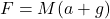Where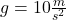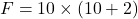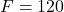N

For finding at what rate motor doing work when it is pulling the block upward,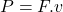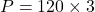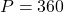W

Therefore, the rate of work is 360 W